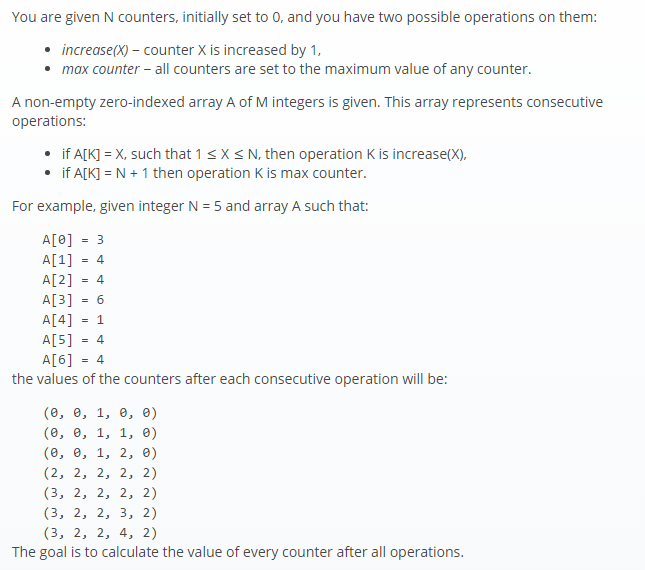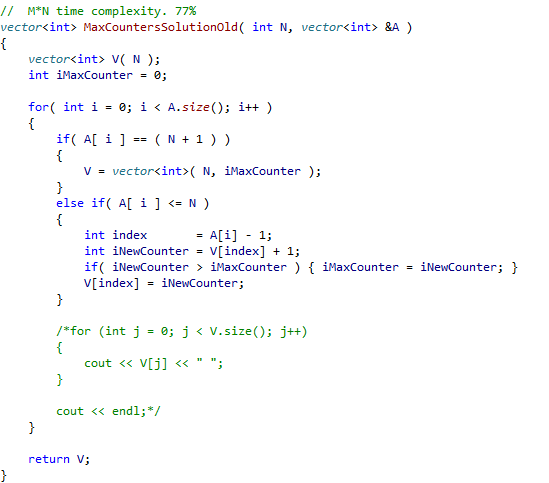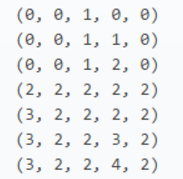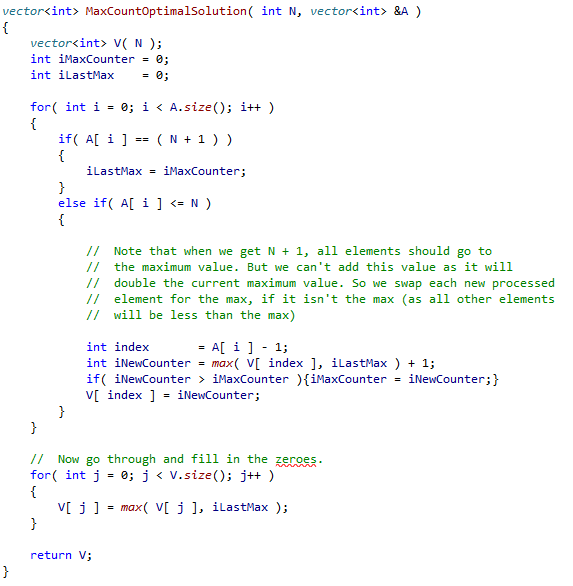### MaxCounters

Programming language: C++The expected time complexity is O(N + M) . N and M run from 1 to 100,000 and each element in A runs from 1 to N+1. In this exercise you must return a vector.

Suboptimal solution: O(N*M)

This simpler yet slower approach will still score at 77%. You loop through the array. If the current element is less than or equal to N, you iterate it, and then take the max of that and the current highest counter. If the element equals the magic value of N + 1, then loop through your array and replace each value with the highest counter value.

Note that calling the constructor vector<int>( N, iMaxCounter ) as below is equivalent to looping through your vector and setting each value to iMaxCounter (and is more efficient). Hence why we get a time complexity of O(N*M).

You can watch the array evolve as in the example, and so this may be a nice way to start before optimising.O(N+M) solution

To get O(N+M) we need to process the N after processing the M. How you may ask? The example diagram of the evolving arrayIs misleading, as we don’t need to get all the steps above, just the final step.  So if we get N+1, we add the current max counter as new array elements are processed, by taking the max of this value and the existing value (this will leave the element with the original max value unchanged, which is what we want).
The only values left will be the zero values that were never updated in our previous loop. We loop through and take the max of these and our previously highest counter.